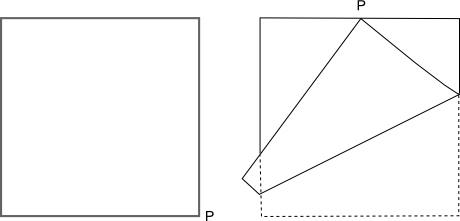#### You may also like### Fitting In

The largest square which fits into a circle is ABCD and EFGH is a square with G and H on the line CD and E and F on the circumference of the circle. Show that AB = 5EF. Similarly the largest equilateral triangle which fits into a circle is LMN and PQR is an equilateral triangle with P and Q on the line LM and R on the circumference of the circle. Show that LM = 3PQ### Look Before You Leap

Can you spot a cunning way to work out the missing length?### Triangle Midpoints

You are only given the three midpoints of the sides of a triangle. How can you construct the original triangle?

# Napkin

##### Age 14 to 16Challenge LevelA square paper napkin is folded so that the corner P coincides with the midpoint of an opposite edge as shown in the diagram.

Investigate the three triangles that are formed by folding in this way (where there is a single thickness of the paper).